## Main

Can quantum features, such as entanglement, survive in the macro domain? This natural question has been with us since the inception of quantum theory. Nowadays, decoherence is widely accepted as one of the fundamental problems limiting the ability of macro systems to maintain quantum features7. As the size of a quantum system increases, it more and more intensively interacts with its surroundings, rapidly destroying its quantum properties. A few technologically demanding experiments1,2,3 have beautifully strengthened this idea. We also know that even if a system is sufficiently well de-coupled from the environment to hold its quantum features, they remain difficult to observe. One of the problems is that decoherence mechanisms inevitably increase the Hilbert space dimension, and even if a small amount of quantumness can survive, the system is no longer well described by the relatively simple theoretical models where measures are known for certifying quantum behaviour. This led, for example, to lively debates8,9,10,11,12 about the presence of entanglement in the experiment reported in ref. 13 where one photon from an entangled pair was phase-covariantly cloned. Measurement precision is another issue. Even if a macro system could be perfectly isolated from its environment, its quantum nature would require extremely precise measurements to be observed14,15.

Following the theoretical proposal presented in ref. 5, we here report on the first experiment where entanglement is created in the micro domain and subsequently displaced to the macro domain, following the spirit of the Schrödinger cat experiment. The displacement is accurately controlled and allows for extending entanglement over unexplored regimes, ranging continuously from a single photon to almost a thousand. Under displacement, the entangled state becomes macroscopic not only because of the large mean photon number but also because its components could be distinguished in a single shot with classical detectors, that is, photon counting detectors coarse-grained by thermal noise, allowing one to resolve only large photon number differences. To check whether entanglement survives in the macro domain, the state is locally displaced back to the micro domain before being detected. This alleviates the problems of detecting macroscopic entanglement because on the one hand, the existence of entanglement can be proved with a well-established entanglement measure operating in the micro domain and on the other hand, the problem of the measurement precision is relegated to the displacement, which can be performed very accurately.

The experiment starts with the heralded creation of a path-entangled state lying in the micro domain (see Fig. 1). A nonlinear optical crystal is pumped by a pulsed laser in the picosecond regime to produce telecom photon pairs by means of spontaneous parametric down-conversion. We set the pump intensity such that the probability of creating a single pair per run is very small. Thus, the detection of one idler photon heralds the creation of a single signal photon. By further placing a narrowband filter before the heralding detector, the signal photons are heralded in spectrally pure states with a bandwidth limited by the pump spectrum. To ensure their spatial purity, they are coupled into a monomode fibre with an efficiency of 50%. The measurement of the second-order auto-correlation g(2)(0) = 1.9(1) unambiguously demonstrates the purity of the signal field16. By sending the heralded photon into a balanced beam splitter, one obtains, leaving aside the loss, a maximally entangled state that describes the two output modes A and B sharing a single photon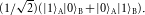This path-entangled state, known as single-photon entanglement, can be seen as the signature of the non-classical feature of the heralded signal photon17,18.

The second step consists of amplifying the mode A by applying a unitary operation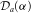corresponding to a displacement in the phase space. The displacement is obtained by combining the mode A and an intense local oscillator on a highly unbalanced beam splitter19,20. The physics behind the displacement is based on an interference process. Hence, the field A and the local oscillator need to be indistinguishable. This is ensured in practice by producing the local oscillator by means of a difference frequency generation (DFG), using an identical nonlinear crystal to the one used for the photon pair creation but stimulated by a continuous-wave telecom laser. The indistinguishability between the resulting local oscillator and the field A is confirmed through a Hong–Ou–Mandel (HOM) type interference21 whose dip, reported in Fig. 2, has a visibility limited only by the reflectivity of the beam splitter and the photon statistics. As such, after the displacement, the detection of an idler photon heralds the generation of an entangled state of the form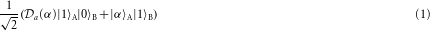|αA results from the displacement of the vacuum, which follows a Poissonian photon number distribution with mean photon number |α|2 equal to the variance.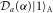represents the displacement of a single photon that is a non-Gaussian state characterized by a photon number distribution with a mean photon number |α|2+1 and a variance 3|α|2. Although |αA anddiffer by only one photon on average, the distance between their photon number distributions is about the square root of their size. Checking whether the photon number falls in the interval [|α|2−|α|,|α|2+|α|] or not, allows one to distinguish their photon number distributions with a probability that increases with the size and approaches 74% in the limit of large |α|. This probability goes up to 90% if the state (1) is seen in another basis so that it readsby dividing the photon number into two intervals [0,|α|2] and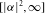(for real positive α). This allows one to distinguish efficiently the two components of the displaced mode with classical detectors, which we now describe. Any measurement of the photon number can be pictured as a pointer on a scale that is shifted when photons are detected (the larger the photon number is, the further the pointer shifts). If the initial state of the pointer is not a delta-function, but has a large spread, for example, due to thermal noise, the probability to distinguish a single photon from the vacuum state in a single shot approaches that of a simple guess. We call such a measurement classical, because it does not resolve the quantum features in the micro domain. On the contrary, the probability to distinguish the states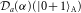and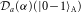in a single shot with such a classical measurement approaches 90% (for large enough α). This makes the state (1) analogous to the famous Schrödinger’s cat state where the dead and alive components can be distinguished without microscopic resolution4.

In the last step of the experiment where we wish to probe whether entanglement survives as its size increases, the displaced state in mode A undergoes another displacement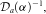which ideally returns it back to the single-photon level. In practice, the de-amplification is realized in a similar manner as the amplification stage but inverting the phase of the local oscillator. The resulting fields can then be probed using single-photon detectors to reveal heralded entanglement between the mode A and B. We emphasize that as the re-displacement is performed locally, it cannot increase the entanglement. A measure of the entanglement after the displacement thus provides a lower bound for the entanglement before the displacement, thus between the micro and macro states.
The state to be measured is described by a density matrix that includes noise and loss. To reveal entanglement, we use the tomographic approach based on single-photon detections presented in ref. 6 and successfully applied in many experiments6,22,23,24. Specifically, from the measured values of the heralded probabilities pm n for detecting m photons in mode A and n in mode B (m,n[0,1]) and of the visibility V of the interference obtained by combining the modes A and B on a balanced beam splitter, a lower bound on the concurrence at the level of the detection is obtained through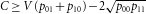. Let us mention that to maximize the observed concurrence, the interference visibility V needs to be maximized and the probability for having one detection in each mode, p11, has to be minimized. This can be done with ease at the single-photon level; however, as the system size increases, this becomes increasingly difficult, as we shall see in the following.
The typical size that can be achieved primarily depends on the precision with which the relative phase of the local oscillators is controlled. This can be intuitively understood in two complementary ways depending on whether the phase noise is regarded as a decoherence mechanism operating on the macro state or as an imprecision of the measurement apparatus. In the first picture, a phase noise acts as if the environment performs a weak measurement of the photon number. (A phase noise, for example, the thermal fluctuations of the fibre length, can be described by a random unitary U(φ) = ${\text{e}}^{i{a}^{†}a\phi }$, where a is the creation operator, that is applied on the state, where the random variable φ follows a normal distribution p(φ). The same transformation can be obtained if the system displaces a pointer state (associated with the environment) |ψ(p)〉E through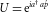. After the environment is traced out such an interaction applies a random phase U(φ) on the system with probability |ψ(φ)E|2 = p(φ). What we are describing is a weak measurement of the photon number by the environmental pointer, initially in a broadened state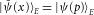.) The weak measurement more easily distinguishes the two macroscopic components when |α| increases, simultaneously degrading the coherence between them. In the second picture, small imperfections in the phase of the re-displacement inevitably add noise in the detected mode. This phase noise decreases the achievable interference visibility V, and simultaneously increases p11 leading rapidly to a zero concurrence. In our set-up, the two local oscillators are produced by splitting the coherent state generated by the DFG process (see Supplementary Information). As presented in Fig. 1, both displacements are made using 90:10 beam splitters to reduce the single-photon losses, and the second unbalanced beam splitter closes a Mach–Zehnder interferometer. To accurately control and tune the relative phase between the two local oscillators, we actively stabilize the interferometer with a feedback loop locked on the side of an interference fringe using a tunable reference laser. We obtain an interference visibility of 99.985(2)%( = 1−ε) that is essentially limited by the path length difference between the two arms (20 μm). This translates into an extinction ratio of 1.5(2)×10−4, meaning that for |α|2 = 6,600(700), a noise of one photon is added into the detected mode. The theoretical model that we derived, which assumes that the relative phase of the two local oscillators is a stochastic variable (following a Gaussian distribution with variance 2ε), that the source produces a two-mode squeezed state, and takes optical loss, detection efficiency, pair production probability into account, predicts a positive concurrence up to |α|2 = 549, which is in excellent agreement with what we observed, as we shall see now.